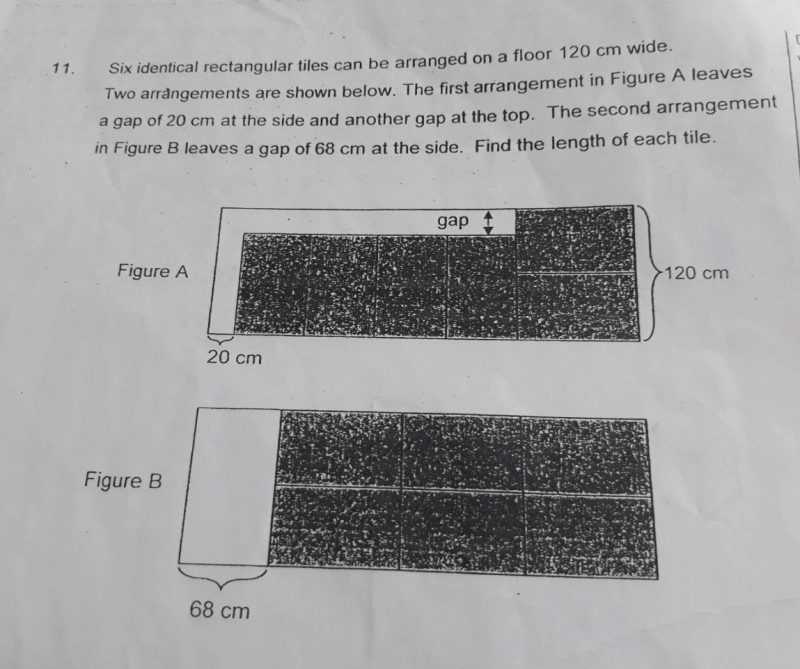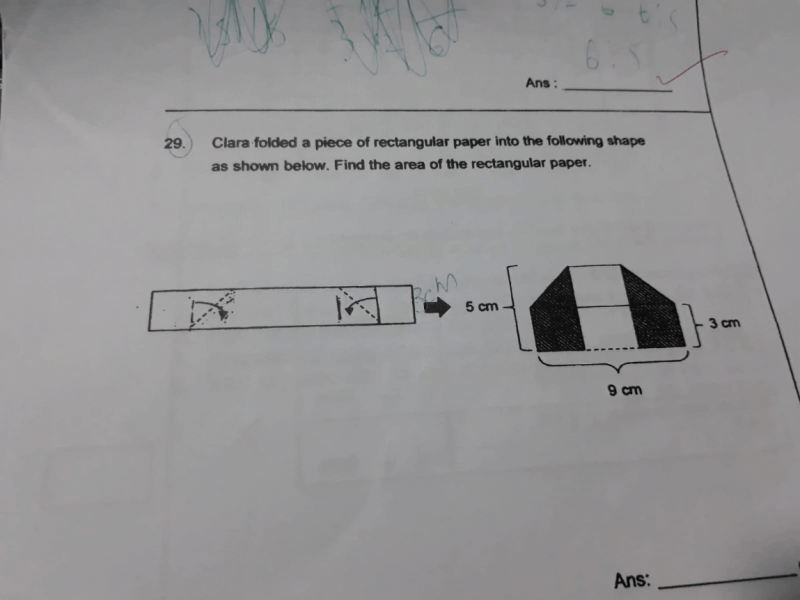# QuestionPlease help me with this question

1 Answer

# Answer

This is similar to this year’s PSLE semi-circle question. We can just treat the length of one rectangular tile as 1 unit. The width of 1 rectangular tile is (120 ÷ 2 = 60) cm as seen from Figure A.

From Figure A, the length of the floor is 20 cm + (60 cm x 4) + 1 unit
= 20 cm + 240 cm + 1 unit
= 260 cm + 1 unit

From Figure B, the length of the floor is 68 cm + 3 units

Since the length of the floor must be the same,

260 cm + 1 unit = 68 cm + 3 units
3 units – 1 unit = 260 cm – 68 cm
2 units = 192 cm
1 unit = 192 cm ÷ 2
= 96 cm

2 Replies 0 Likes

Thank you very much for explaining

0 Replies 0 LikesCan you please help me with this question ?

0 Replies 0 Likes